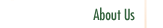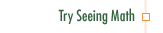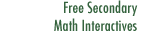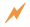Systems of Linear Equations

Participants define a linear function in two variables that is expressed in the form Ax+By = C. They then compare the graphs of two or more functions of this form, and explore graphically how some, but not all, algebraic operations leave the solution set of the system unchanged. Participants also look at traditional methods of solving systems of linear equations by substitution and by combination.

Professional Development Goals

• Use the function approach to understand how graphic and algebraic methods relate when solving systems of equations
• Explore the effects of algebraic operations on solution sets, using either individual linear functions or pairs
• Use graphic representations to model real world applications of systems of linear functionsClick to Get Seeing Math™ SecondaryCourse Listing Proportional Reasoning Linear Functions Transformations of Linear Functions Linear Equations Systems of Linear Equations Quadratic Functions Transformations of Quadratic Functions Quadratic Equations Data Analysis